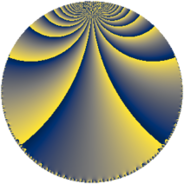# Properties

 Label 304.5.jLevel $304$ Weight $5$ Character orbit 304.j Rep. character $\chi_{304}(37,\cdot)$ Character field $\Q(\zeta_{4})$ Dimension $316$ Sturm bound $200$

# Related objects

## Defining parameters

 Level: $$N$$ $$=$$ $$304 = 2^{4} \cdot 19$$ Weight: $$k$$ $$=$$ $$5$$ Character orbit: $$[\chi]$$ $$=$$ 304.j (of order $$4$$ and degree $$2$$) Character conductor: $$\operatorname{cond}(\chi)$$ $$=$$ $$304$$ Character field: $$\Q(i)$$ Sturm bound: $$200$$

## Dimensions

The following table gives the dimensions of various subspaces of $$M_{5}(304, [\chi])$$.

Total New Old
Modular forms 324 324 0
Cusp forms 316 316 0
Eisenstein series 8 8 0

## Trace form

 $$316q - 4q^{4} - 4q^{5} - 4q^{6} + O(q^{10})$$ $$316q - 4q^{4} - 4q^{5} - 4q^{6} - 4q^{11} - 956q^{16} - 8q^{17} - 706q^{19} - 2608q^{20} + 3124q^{24} - 4768q^{26} + 3840q^{28} + 2216q^{30} - 2688q^{35} + 7068q^{36} + 208q^{38} + 16980q^{42} - 4q^{43} - 1612q^{44} + 2820q^{45} - 8q^{47} - 100164q^{49} - 5216q^{54} - 8992q^{58} + 7548q^{61} + 15612q^{62} + 19200q^{63} + 23552q^{64} + 5236q^{66} - 11648q^{68} + 28808q^{74} - 27836q^{76} + 9600q^{77} + 17072q^{80} - 207044q^{81} + 9132q^{82} - 24004q^{83} - 24904q^{85} + 9336q^{92} + 320q^{93} + 25340q^{95} - 98640q^{96} + 3268q^{99} + O(q^{100})$$

## Decomposition of $$S_{5}^{\mathrm{new}}(304, [\chi])$$ into newform subspaces

The newforms in this space have not yet been added to the LMFDB.